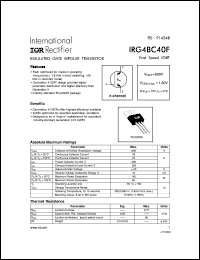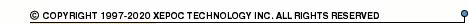More than12 564 936 queries processedIRG4BC40 series datasheets. Manufacturer: International Rectifier.

 IRG4BC40 Insulated gate bipolar transistor. VCES = 600V, VCE(on)typ. = 1.50V @ VGE = 15V, IC = 27A in 3-pin TO-220AB package. Operational temperature range from -55°C to 150°C. Datasheet*) IRG4BC40K Insulated gate bipolar transistor. VCES = 600V, VCE(on)typ. = 2.1V @ VGE = 15V, IC = 25A in 3-pin TO-220AB package. Operational temperature range from -55°C to 150°C. Datasheet*) IRG4BC40S Insulated gate bipolar transistor. VCES = 600V, VCE(on)typ. = 1.32V @ VGE = 15V, IC = 31A in 3-pin TO-220AB package. Operational temperature range from -55°C to 150°C. Datasheet*) IRG4BC40U Insulated gate bipolar transistor. VCES = 600V, VCE(on)typ. = 1.72V @ VGE = 15V, IC = 20A in 3-pin TO-220AB package. Operational temperature range from -55°C to 150°C. Datasheet*) IRG4BC40W Insulated gate bipolar transistor. VCES = 600V, VCE(on)typ. = 2.05V @ VGE = 15V, IC = 20A in 3-pin TO-220AB package. Operational temperature range from -55°C to 150°C. Datasheet*) IRG4BC40F INSULATED GATE BIPOLAR TRANSISOR(Vces=600V, Vce(on)typ.=1.50V, @Vge=15V, Ic=27A) Datasheet*)